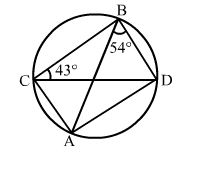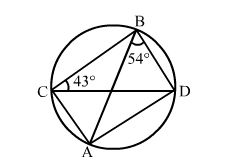# In the given figure, ∠ABD = 54° and ∠BCD = 43°, calculate

Question:

In the given figure, ABD = 54° and ∠BCD = 43°, calculate

(i) ∠ACD

(iii) ∠BDA.Solution:(i)
We know that the angles in the same segment of a circle are equal.
i.e., ABD = ACD = 54°

(ii)
We know that the angles in the same segment of a circle are equal.
i.e., BAD = BCD = 43°

(iii)
In ΔABDwe have:
BAD + ADB + DBA = 180°  (Angle sum property of a triangle)
⇒ 43° + ADB + 54° = 180°
⇒ ADB = (180° – 97°) = 83°
⇒ BDA = 83°